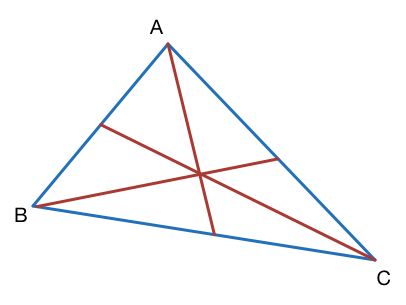# Triangle of MediansTriangle $ABC$ has sides of length 24, 35, and 53.

Triangle $DEF$ has side lengths equal to the lengths of the three medians of triangle $ABC$.

Find the area of triangle $DEF.$

×